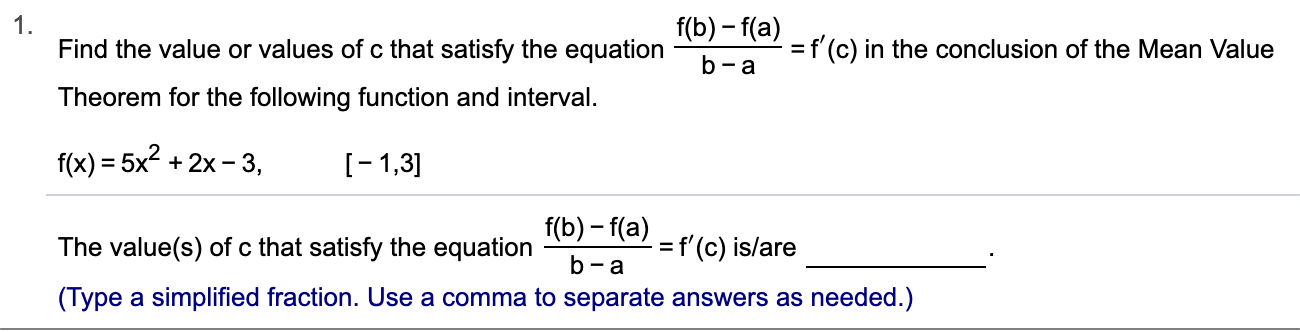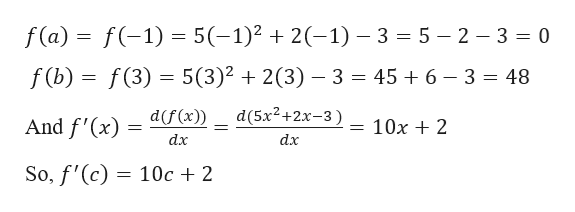# 1Find the value or values of c that satisfy the equationf(b)f(a)=f(c) in the conclusion of the Mean Valueb-aTheorem for the following function and intervalf(x) 5x2x-3-1,3f(b)-f(a)=f'(c) is/areThe value(s) of c that satisfy the equationb-a(Type a simplified fraction. Use a comma to separate answers as needed.)

Question
2 views

Can you help me step by step?help_outlineImage Transcriptionclose1 Find the value or values of c that satisfy the equation f(b)f(a) =f(c) in the conclusion of the Mean Value b-a Theorem for the following function and interval f(x) 5x2x-3 -1,3 f(b)-f(a) =f'(c) is/are The value(s) of c that satisfy the equation b-a (Type a simplified fraction. Use a comma to separate answers as needed.) fullscreen
check_circle

Step 1

Given,

Step 2

Here, a = -1 and b = 3

Now,help_outlineImage Transcriptionclosef(a) f(-1)= 5(-1) 2(-1) 3 5 2 3 0 2(3) - 3 = 45 + 6 - 3 = 48 f (b) f(3) 5(3)2 d(f (x)) d(5x2+2x-3) And f'(x) = 10x2 11 dx dx So, f'(c) 10c2 fullscreen
Step 3

Now, applying the Mean...

### Want to see the full answer?

See Solution

#### Want to see this answer and more?

Solutions are written by subject experts who are available 24/7. Questions are typically answered within 1 hour.*

See Solution
*Response times may vary by subject and question.
Tagged in

### Derivative